# Calculus Riemann sum Questions

1.

Which of the following sums does not equal the others? (4 points)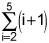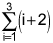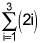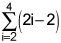2.

Estimate the area under the curve f(x) = x2 from x = 1 to x = 5 by using four inscribed (under the curve) rectangles. Answer to the nearest integer. (4 points)

3.

List x1, x2, x3, x4 where xi is the right endpoint of the four equal intervals used to estimate the area under the curve of f(x) between x = 3 and x = 5. (4 points)

 3, 3.5, 4, 4.5 3, 3.2, 4.6, 5 3.5, 4, 4.5, 5 3.25, 3.75, 4.25, 4.75

4.

Write the summation to estimate the area under the curve y = 2x2 + 1 from x = 0 to x = 4 using 4 rectangles and left endpoints. (4 points)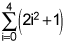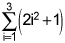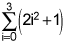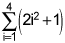5.

If the area under the curve of f(x) = x2 + 2 from x = 1 to x = 6 is estimated using five approximating rectangles and right endpoints, will the estimate be an underestimate or overestimate? (4 points)

 Underestimate Overestimate The area will be exact The area cannot be estimated with just five rectangles

1.

The Riemann sum,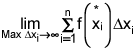, is equivalent to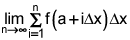with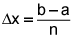.

Write the integral that produces the same value as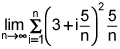. (4 points)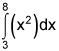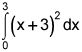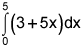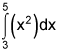2.

Write the Riemann sum to find the area under the graph of the function f(x) = x3 from x = 2 to x = 5. (4 points)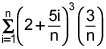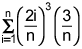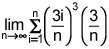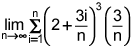3.

Use your calculator to evaluate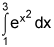. Give your answer to the nearest integer. (4 points)

4.

Use geometry to evaluate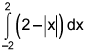. (4 points)

 2 4 8 12

5.

Use geometry to evaluate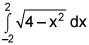. (4 points)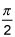π 2π Cannot be evaluated

1.

Given that the antiderivative of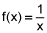is F(x) = Ln|x| + C, evaluate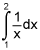. (4 points)

 ln3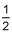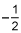ln2

2.

Evaluate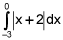. (4 points)

 -2.5 1.5 2.5 3

3.

Given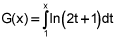, find G ‘(x). (4 points)

 ln(2x + 1) ln(2x + 1) – ln3 ln(2t + 1) + C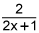4.

Find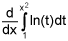. (4 points)

 2xln(x2)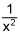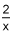ln(x2)

5.

Determine the interval on which f(x) = ln(x) is integrable. (4 points)

 (0, ∞) [0, ∞) (-∞, 0) U (0, ∞) All reals